# Lecture 10 - Planetary Temperatures (10/15/98)Light --- | --- AtmospheresChapter 2-1, 8-1, 8-6, P6-3 (ZG4)

Hurricane Fran approaching the Bahamas in September 1996, as viewed by GOES-8 (NASA).
Key Question: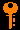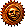## Investigations:

• How does the color of light emission from a hot solid object depend upon the temperature?
• What is the Kelvin temperature scale?
• How does the wavelength of the maximum brightness depend upon the temperature (Wien's Law)?
• How do we determine the surface temperature of the Sun?
• How does the surface flux emitted by a hot body depend upon the temperature (Stefan-Boltzmann Law)?
• Why did Planck introduce quantization of light into the blackbody formulation?
• What is a blackbody?
• What is Planck's blackbody function?
2. Thermal Equilibrium
• How does a blackbody come into equilibrium with radiation?
• At what temperature will a blackbody be at equilibrium with radiation flux F?
• What do we mean by sub-solar temperature?
• What do we calculate for the sub-solar temperature at the Earth's orbit of 1 AU from the Sun?
• Why is this too high?
3. Greenhouse Effect
• What is the difference between a planet's albedo and its greenhouse factor?
• Why is the surface of Venus around 700K, while the cloud tops are at the expected temperature of 330K?

It is well-known that if you heat stuff up, it glows! If you turn the burner on your electric stove to high, it starts to glow red. A flame is bright, and burning coals glow red. If you've ever seen iron or steel being heated in a furnace, you know that as you heat it to higher and higher temperatures it glows red, yellow, bluish, then white-hot, and at the same time brighter and brighter.

There is obviously a connection with temperature and light emission. The hotter something is, the brighter it glows, and the "bluer" a color the light that it emits is.

The temperature of a body is a measure of how fast the atoms and molecules in it are jiggling about. Higher temperature means higher average velocity (actually, average squared-velocity). In physics, the temperature is measured using a scale called the Kelvin scale (K). This is the same degrees in the centigrade or Celsius scale (C) (a change of 1 K = 1 C), but measured from absolute zero (zero velocity, 0 K = -273 C = -460 F) instead of from the freezing of water ( 0 Celsius = 273 Kelvin = 32 F). Thus, water boils at 373 K (100 C = 212 F).

The kinetic energy of a particle is proportional to the square of the velocity:

E = m v^2 / 2

If you as what the distribution of kinetic energies of atoms in a gas at a temperature T is, you find a distribution that has is peaked at a characteristic kinetic energy, with few atoms at low energies (velocities), and few atoms at high energies (velocities). The average kinetic energy (we denote an average some quantity X by < X >):

< E > = < mv^2/2 > = 3kT/2

where k is Boltzmann's constant (k=8.6 x 10^-5 eV/K).

Because the electric force is transmitted by photons, then it turns out that whenever you apply a force to an electron, and it is accelerated, it will emit a photon. The fact that accelerating electrons always emit radiation is an important one in astonomy, and we will return to it more than once. At this point, it is important in that collisions between atoms can cause the electrons in the outer shells to accelerate, and thus emit radiation. The spectrum of this "thermal" radiation depends on the thermal distribution of velocities and thus kinetic energies of the atoms in the material.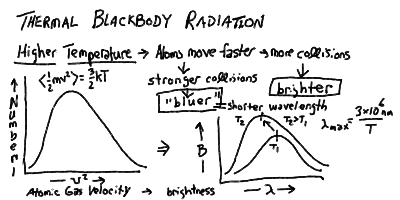If a material is opaque, that is if nearly all the photons emitted by an atom inside the matter will be absorbed and re-emitted by other atoms in the substance before escaping out through the surface, then the energy of the radiation will come to equilibrium with the energy in the thermal motions of the agitated electrons. Thus, opaque matter at a temperature T (in Kelvin) radiates a continuous spectrum, whose peak shift to shorter wavelength and total energy rise with the temperature: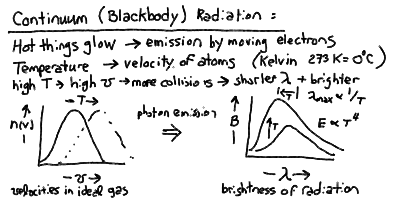The peak wavelength of the blackbody radiation is inversely proportional to the temperature, and the total power radiated per square meter of surface is proportional to the fourth power of the temperature: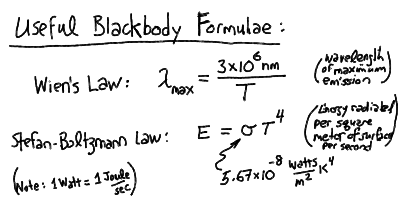Thus, we can relate the observed spectra and radiative fluxes to the temperature of a blackbody. Most objects (stars, planets, etc) have an approximately blackbody spectrum. Therefore, this is one of the most important concepts in astrophysics.

## Example Problem: Temperature of Mercury

An example of the use of thermal equilibrium with blackbody radiation is the calculation of the mean temperature of the planet Mercury, which has an orbital semi-major axis a=0.39 AU, and an albedo of A=0.06 (similar to the moon). As Mercury has no atmosphere to speak of, there is no greenhouse factor G=0 as it is just bare rock!

First we calculate the subsolar temperature at 0.39 AU:

Tss = [ Lsun / (4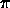r2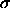) ]1/4
= 394 K [ r / 1 AU ]-1/2 = 631 K

and thus the equilibrium temperature averaged over Mercury's sphere is

Teq = Tss 2-1/2 [ (1-A) / (1-G) ]1/4
= 631 K 2-1/2 [ 0.94 ]1/4 = 439 K.

This should represent the average temperature, but as Mercury has no atmosphere to distribute the heat, and the rock of the planet is a (relatively) poor conductor, it should range all the way up to the sub-solar temperature of 631 K at the sub-solar point where the Sun is on the zenith. In fact, since the eccentricity of Mercury's orbit is e=0.206, and so the perihelion distance is

rp = a ( 1 - e ) = 0.39(1-0.206) = 0.31 AU

and the sub-solar temperature at perihelion should be

Tss,p = 394 K [ 0.31 ]-1/2 = 708 K.

The measured temperatures (from the Mariner missions) range from 100 K on the dark side, to 700 K on the bright side, exactly in line with what we find using these simple calculations!

## Blackbody Radiation - Some Web ResourcesPrev Lecture ---Next Lecture ---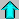Astr11 Index ---Astr11 Home

smyers@nrao.edu Steven T. Myers